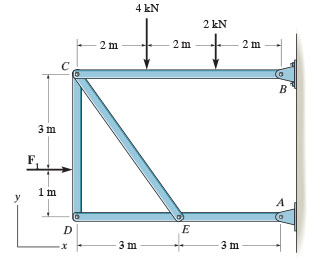# 4 kN 2 kN 2m 2m 2m 3 m 1 m A E 3 m 3 m x

Question

Consider the frame shown in (Figure 1). Suppose that F1 = 8 kN . Determine the x and y components of the force that the pin at B exerts on the frame using scalar notation. Determine the x and y components of the force that the pin at A exerts on the frame using scalar notation.help_outlineImage Transcriptionclose4 kN 2 kN 2m 2m 2m 3 m 1 m A E 3 m 3 m x fullscreen